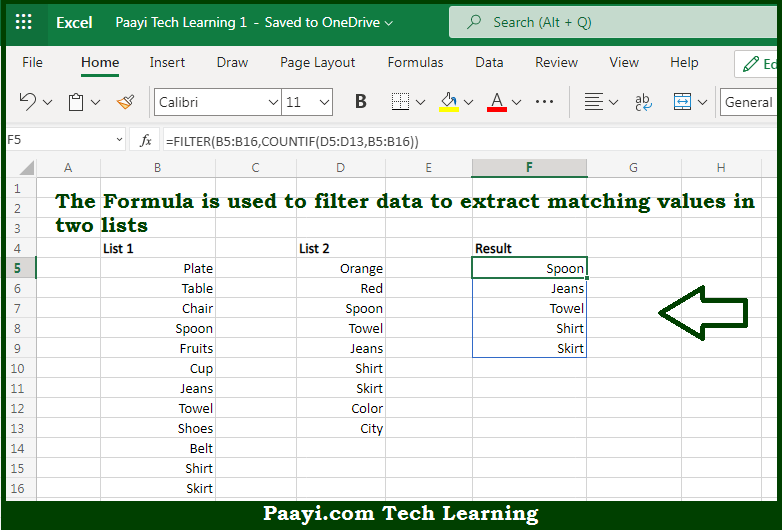# Learn How to Filter to Extract Matching Values in Microsoft Excel

Written by | 0 Comments | 550 Views

In this article, you will learn how to evaluate things in Dynamic Arrays with formulas in Microsoft Excel using a single/combination(s) of functions. You will also know How to Filter to Extract Matching Values and see the generic formula.

Learn How to Filter to Extract Matching Values in Microsoft Excel

The main purpose of this formula is to extract matching values in two lists. Here we will learn how to filter to extract matching values in the given data range in the workbook in Microsoft Excel. That implies, with the help of a formula based on the FILTER, COUNTIF, or COUNTIFS function you can able to extract matching values in two lists. So, with the help of this formula, you can able to extract matching values in the given data range in the workbook in Microsoft Excel.

General Formula to Filter to Extract Matching Values

=FILTER(list1,COUNTIF(list2,list1))

The Explanation for the Filter to Extract Matching ValuesSo we know that with the help of the given formula above you can able to extract matching values in two lists. Here we will learn how to filter to extract matching values in the given data range in the workbook in Microsoft Excel. As we know that the formula relies on the FILTER function to retrieve data based on a logical test built with the COUNTIF function. So, with the help of this formula, you can able to extract matching values in two lists. Here we will learn how to filter to extract matching values in the given data range in the workbook in Microsoft Excel.